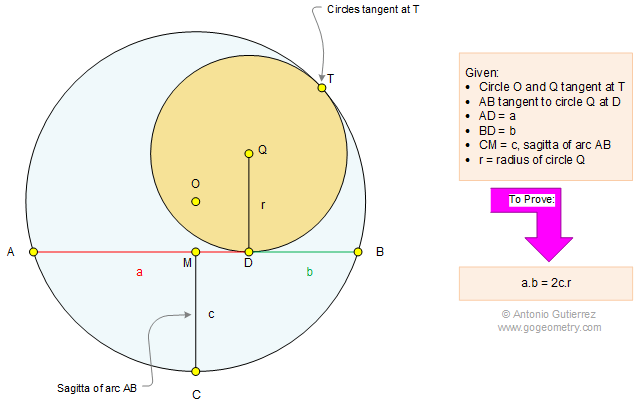# Problem 1094: Tangent Circles, Tangent Chord, Radius, Sagitta of Arc. Level: High School, SAT Prep, College Geometry

< PREVIOUS PROBLEM  |  NEXT PROBLEM >

 The figure shows the circles O and Q tangent at T. Chord AB is tangent to circle Q at D, AD = a and BD = b. The sagitta of arc AB is CM = c. If r is the radius of circle Q, prove that a.b = 2c.rHome | Search | Geometry | Problems | All Problems | Open Problems | Visual Index | 1091-1100 | Circle | Tangent Line | Tangent Circles | Chord | Sagitta, Definition | Sagitta problems | Email | Post a comment or solution | By Antonio GutierrezLast updated: Mar 8, 2015